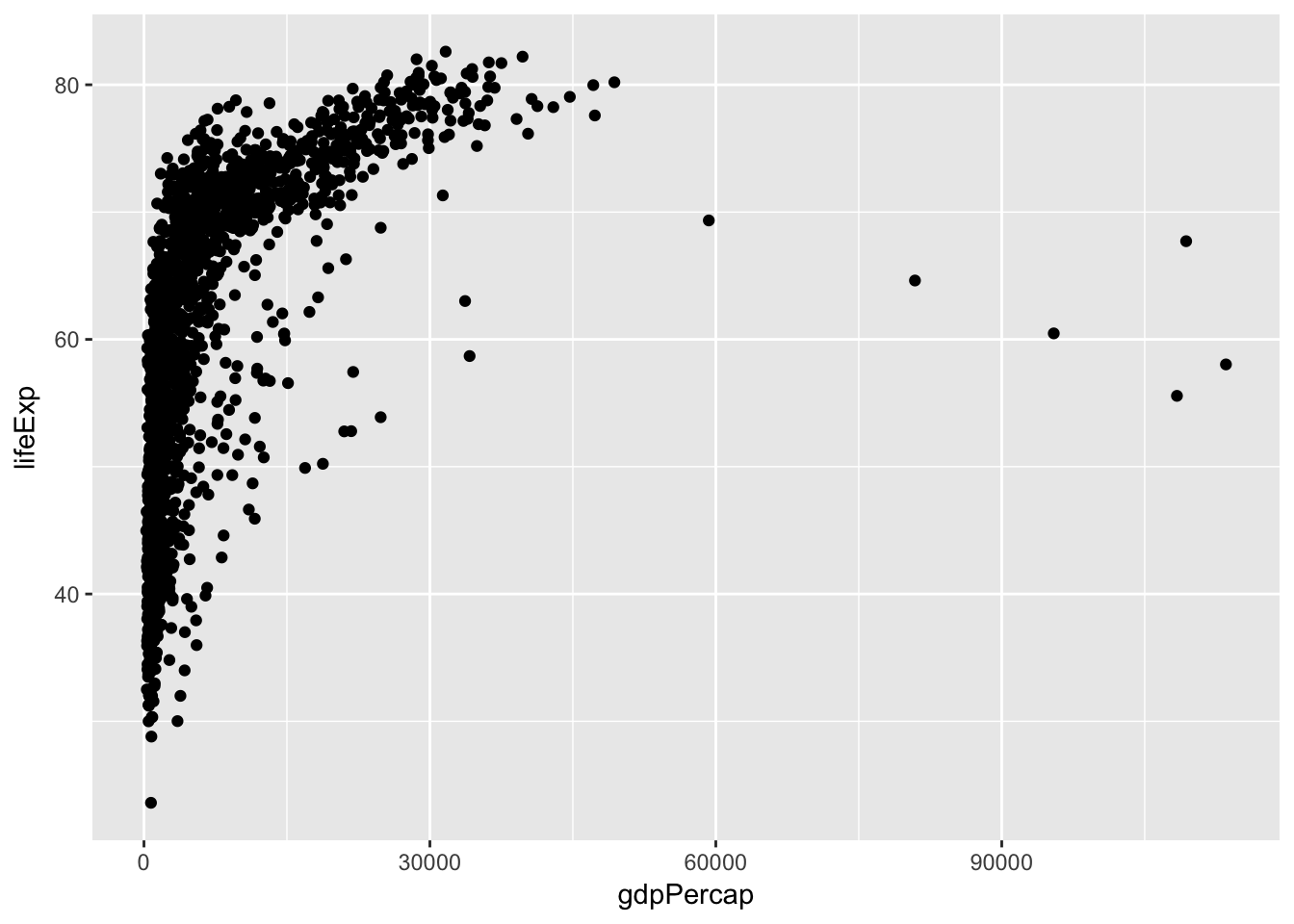### Preparations

There are several different systems for creating data visualizations in R. We will introduce ggplot2, which is based on Leland Wilkinson’s Grammar of Graphics. The learning curve is a bit steep, but ultimately you’ll be able to produce complex graphs more quickly and easily.

You first need to install the ggplot2 package:

``install.packages("ggplot2")``

You then need to load the package:

``library(ggplot2)``

We’ll consider the gapminder data from the last lesson. If it’s not within your R workspace, load it again with `read.csv`.

``gapminder <- read.csv("http://kbroman.org/datacarp/gapminder.csv")``

### A first plot

An initial bit of code, to make a scatterplot:

``ggplot(gapminder, aes(x=gdpPercap, y=lifeExp)) + geom_point()``Two key concepts in the grammar of graphics: aesthetics map features of the data (for example, the `lifeExp` variable) to features of the visualization (for example, the y-axis coordinate), and geoms concern what actually gets plotted (here, each data point becomes a point in the plot).

Another key aspect of ggplot2: the `ggplot()` function creates a graphics object; additional controls are added with the `+` operator. The actual plot is made when the object is printed.

The following is equivalent to the code above. The actual plot isn’t created until the `p2` object is printed. (When you type an object’s name at the R prompt, it gets printed, and that’s the usual way that these plots get created.)

``````p1 <- ggplot(gapminder, aes(x=gdpPercap, y=lifeExp))
p2 <- p1 + geom_point()
print(p2)``````

It’s best to do the x-axis on a log scale.

``ggplot(gapminder, aes(x=gdpPercap, y=lifeExp)) + geom_point() + scale_x_log10()``